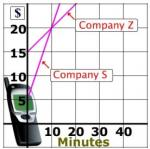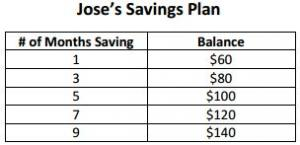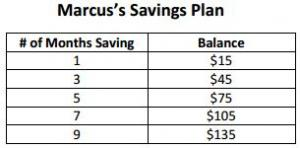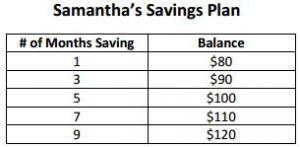# Let's Get Started

Let's investigate how a system of equations applies to real world situations, and what the solution represents.

TEKS Standards and Student Expectations

A(5) Linear functions, equations, and inequalities. The student applies the mathematical process standards to solve, with and without technology, linear equations and evaluate the reasonableness of their solutions. The student is expected to:

A(5)(C) solve systems of two linear equations with two variables for mathematical and real-world problems

Resource Objective(s)

Students will use a variety of methods to find the solution to a system of equations. They will determine the reasonableness of a solution in a real world problem.

Essential Questions

What types of real world situations can be represented by a system of equations?

How can substitution be used to solve a system of equations?

How can a graph be used to determine the solution to a system of equations?

How can you determine if a solution is reasonable?

Vocabulary

# Systems of Equations and Solutions

A system of linear equations is two or more linear equations that use two or more variables.

A solution to a system of linear equations is a pair of numbers for x and y that make both equations true.

To find a solution to a system of linear equations, one of three methods can be used: substitution, elimination, or graphing.

To determine if a solution is reasonable, verify whether the pair of values satisfies the conditions given in the verbal situation.

Example

Iris enjoys making friendship bracelets. She is able to braid a purple bracelet at a rate of 5 cm per day, and a green bracelet at a rate of 4 cm per day. The purple bracelet is already 5 cm long, and the green bracelet is already 11 cm long. Iris estimates that the two bracelets would be the same length after four days. Is her estimate correct?Follow the steps to determine if the estimate is reasonable.

To determine the number of days needed for the bracelets to reach the same length you can solve by substitution.

Solve by substitution:

From this we that the bracelets would be the same length after 6 days.

Purple bracelet: y = 5(6) + 5 = 35 cm.
Green bracelet: = 4(6) + 11 = 35 cm.
The bracelets would each be 35 cm after 6 days.

Read each of the following questions, and determine if the proposed solution is reasonable.

# Systems of Linear Equations: Real World Applications

A system of equations can also be solved by elimination. In this method, you add or subtract the equations in order to eliminate one of the variables, and solve for the other variable.

Example 1

A local department store sells a bag of 30 mini candy bars and 20 snack-size candy bars for $7. They will also sell a bag of 10 mini candy bars and 50 snack-size candy bars for$11. Marla estimated that in both bags, the mini candy bars cost $0.10 each, and the snack-size candy bars cost$0.20 each. Was Marla's estimate reasonable?

Follow the steps below to solve the system of equations by elimination, and determine if the estimate is reasonable.

Systems of equations can also be represented in a graph. In this case, the intersection shows the solution.

Example 2

The following graph shows the cost of two different cell phone plans from two different companies.# Linear Equations and Linear Systems in the Real World

A set of tables can also be used to show a system of equations. In this case, it is helpful to extend the table to find missing pieces of data.

The tables below show the amount of money that three friends have saved over a period of 9 months.# Break Even Points

A system of equations can also be used to find break even points. In these problems there is specific amount of money that has been invested in a product, and a certain amount that the product is being sold for in order to try and make a profit. When finding the break even point you are looking for the point where the money invested and the profit are equivalent.

Example

Joe's Cookie Stand pays $480 for rent. They incur an expense of$0.08 per cookie. They sell the cookies for \$0.32 each. How many cookies do they have to sell to break even?

Read each of the following questions to determine if the proposed solution is reasonable.